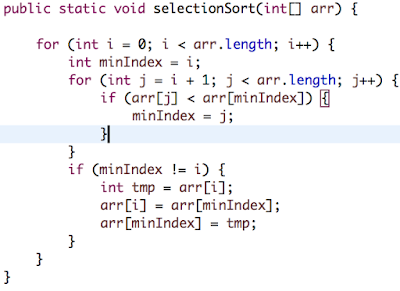Showing posts with label Selection sort. Show all posts
Showing posts with label Selection sort. Show all posts## Selection sort

 Selection sort is conceptually the most simplest sorting algorithm. This algorithm will first find the smallest element in the array and swap it with the element in the first position, then it will find the second smallest element and swap it with the element in the second position, and it will keep on doing this until the entire array is sorted. It is called selection sort because it repeatedly selects the next-smallest element and swaps it into the right place.

```public class SelectionSort {

public static void main(String[] args) {

int[] arr = { 4, 14, 6, 15, 8, 0, 313, 80, 21, 67, 81, 142 };

printArray(arr, "BEFORE SORTING : ");

selectionSort(arr);

printArray(arr, "AFTER SORTING : ");
}

public static void selectionSort(int[] arr) {

for (int i = 0; i < arr.length; i++) {
int minIndex = i;
for (int j = i + 1; j < arr.length; j++) {
if (arr[j] < arr[minIndex]) {
minIndex = j;
}
}
if (minIndex != i) {
int tmp = arr[i];
arr[i] = arr[minIndex];
arr[minIndex] = tmp;
}
}
}

public static void printArray(int[] arr, String msg) {
System.out.print(msg);
for (int i : arr) {
System.out.print(i + ", ");
}
System.out.println();
}
}
```

OUTPUT:

```BEFORE SORTING : 4, 14, 6, 15, 8, 0, 313, 80, 21, 67, 81, 142,
AFTER SORTING : 0, 4, 6, 8, 14, 15, 21, 67, 80, 81, 142, 313,
```## Selection sort in Java

 Selection sort is a combination of searching (search for smaller element) and sorting (Swap with first element) and move on. During each pass unsorted element with the smallest value will be swapped to its proper position. Number of times the loop passes through the array will be one less than the size of array (N-1 times).  In selection sort we need to 2 loops as same as bubble sort, where here inner loop will find the smallest element and outer loop need to swap with the other element and need to place it in proper position. Now lets see simple Java code for selection sort.

```public class SelectionSort {

public static void main(String[] args) {
int array[] = {3,4,8,5,1,7,9,2,6};
System.out.print("Array before sorting : ");
printArray(array);

selectionSort(array);

System.out.print("Array after sorting : ");
printArray(array);
}

public static void selectionSort(int[] values) {
for (int i = values.length - 1; i > 0; i--) {
int first = 0;
for (int j = 1; j <= i; j++) {
if (values[j] > values[first])
first = j;
}
int tmp = values[first];
values[first] = values[i];
values[i] = tmp;
}
}

public static void printArray(int[] array) {
for (int i : array) {
System.out.print(i + ", ");
}
System.out.println();
}
}
```

OUTPUT:

```Array before sorting : 3, 4, 8, 5, 1, 7, 9, 2, 6,
Array after sorting : 1, 2, 3, 4, 5, 6, 7, 8, 9,
```